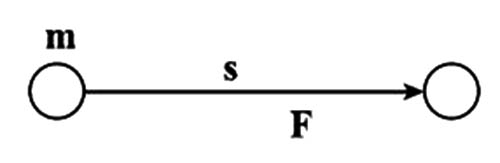Physics

# What is Kinetic Energy?

We sometimes notice that cricket ball hits the stamp and strikes it down. If anything hard hits the glass window the glass breaks down. If we throw stones at mango or jujube it may fall down.

From the above example, we can see energy exists in the body in motion. The capacity of doing work acquired by a moving body due to its motion is called kinetic energy.

Why does the object displace from its initial position? This is because the hit makes the pen move and the pen obtains the ability to work that is kinetic energy is produced in it. So it could displace the object.

Creating velocity in a body in rest or increasing the velocity of a moving object means to produce acceleration in it. For this force has to be applied. As a result, work will be done on the body. For this, the body will obtain the ability to work and this work will be stored in the object as kinetic energy. This is why all moving objects are in possession of kinetic energy. The body will be able to perform this amount of work before it rests. Let a force F be applied to a body of mass m at rest.

The body attains a velocity v. Suppose the body moves a distance s in the direction of the force. The work done to produce this velocity of the body is its kinetic energy.

Therefore, Kinetic energy = Work done

= Force x displacement = F x s

Or, Ek = mas; [ as, F = ma]But, v2 = u2 + 2as

or, as = v2 / 7; [since, initial velocity, u = 0]

so, Ek = ½  mv2

Kinetic energy = ½  x mass x (velocity)2